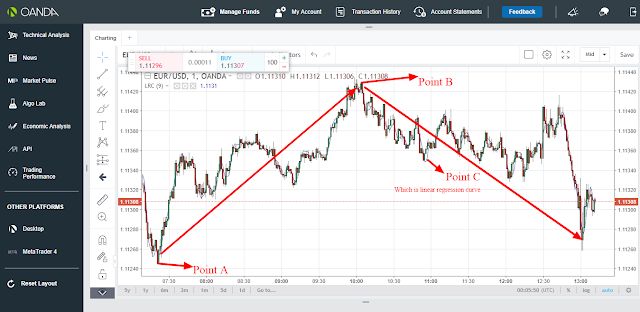Linear regression curve is a trend following indicator.

Being a trend following indicator,Linear regression curve was created to enable traders know whether the market  is in an uptrend or a downtrend. Just like other trend following indicator,Linear regression curve is also based on support and resistance.

Linear regression curve is derived based on linear regression line equation .The equation will produce a straight line when fitted with various prices over a given period.

Therefore,the values of linear regression curve which follows the linear equation rule is calculated as follows;

### y= a +bx

where as

y is price

x is period

b is constant

Since linear regression curve is a trend following indicator,it therefore follows that when the market is moving upwards, the linear regression curve will also be moving upwards while when the market is moving downwards the linear regression curve will also be trending downwards.

Based on support and resistance, when the price rises above the linear regression curve when the market and the linear regression curve are in an uptrend, a downward reversal movement will take place and the market and the linear regression curve will now be trending downwards thus signaling the trader to be trading in a downwards market.

On the other hand when the price falls below the linear regression curve when the market and the linear regression curve are in a downwards trend, an upward reversal movement  will take place and the market and the linear regression curve will now be trending upwards thus signaling the trader to be trading in an upward market. This is indicated as from the candle sticks chart below;From the candle sticks chart above, there are 3 points, point A, B and C. Point A and B are the support and resistance point while point C which is a blue curve represents the linear regression curve.

At point A the market and the linear regression were initially moving downwards. The price in the market then falls below linear regression curve thus resulting to an upward reversal movement for both the market and the linear regression curve. This will signal the trader to close any sell position position and open a buy position.

On the other hand, at point B the market and the linear regression were initially moving upwards.The price in the market then rises above the linear regression curve thus resulting to a downward reversal movement for both the market and the linear regression curve.This will signal the trader to close any buy position and open a sell position.

### Recommendation:If you are a day trader just use 1 min, 5 min, 15 min and 30 min timeframe while if you are a swing trader just use 1 hour and above timeframe if you want linear regression curve indicator to work well for you.

You are always free to support our work from below;

skrill donation:[email protected]

Neteller donation:[email protected]

Ethereum; 0x346570c491b76c7cd51699bdd272762111b743a9

Litecoin: LeEH7B82ccXJ6QoibCryGJNpDGNx2x77fL

Dogecoin: DLShs2vqhMc1ggqo8MPPMBTcPAZdqCZoWd

lightning network:tipping.me:https://tippin.me/@quintomudigo